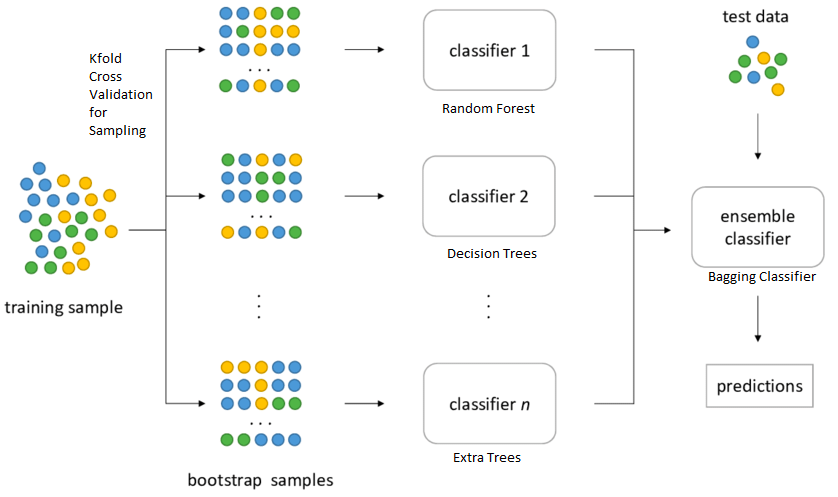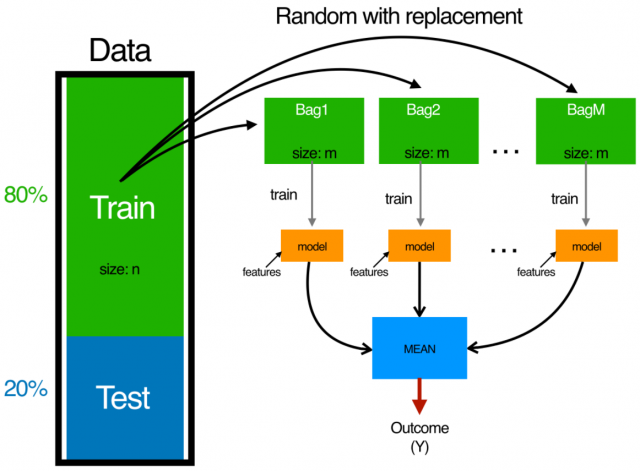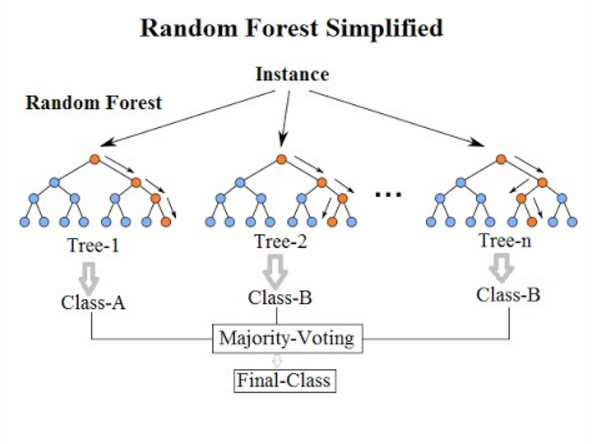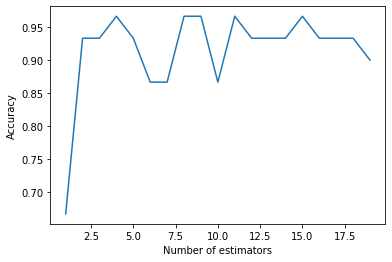# Bagging Classification

## Introduction

To understand bagging classification, we first need to understand the ensemble techniques. Ensemble means combining multiple models. This method trains various models with different datasets and gets the output. Ensemble techniques are of three types: bagging, boosting, and stacking; today, we will study bagging classification.

## What is Bagging Classification?

Initially, let us assume a particular problem statement has a dataset with n number of rows. Now will create multiple base models m and provide a sample of data for training the specific model. Note that the dataset provided to various models is always less than the original dataset, i.e., n. Also, the dataset provided is not the same for all the models; the original dataset is resampled to train the next model.For example: Let us assume

Original dataset: 1,2,3,4,5,6

Then the dataset provided to various models will be row-wise resampled and hence will look like:

Resampled data 1: 6,3,2,5,1,4

Resampled data 2: 2,4,1,3,6,5

Resampled data 3: 4,1,3,6,5,2

Resampled data 4: 3,1,5,2,6,4

Resampled data 5: 5,2,1,3,6,4

The final result will be calculated based on voting or by calculating the mean of all the results of the respective models.

The bagging classification technique is also known as bootstrap aggregation.### Uses of Bagging Classification

Bagging Classification is most commonly used in Random Forest models, be it classification or regression. In bagging classification, we have various base learners models; therefore, the base models are decision trees in a random forest. So to train the decision trees, feature sampling and row sampling with replacement are done. Once all the decision trees are trained on the replaced sampled data, they produce the required output or prediction based on a majority vote. Although, in regression, the final result is calculated by taking a mean of all the outcomes of the decision trees.• It improves the accuracy and stability of machine learning models.
• It reduces the variance hence avoiding overfitting.
• Bagging classification is a particular case of the average model approach.

## Implementation of bagging classification

``````#importing necessary libraries
import matplotlib.pyplot as plt
from sklearn.tree import DecisionTreeClassifier
from sklearn.ensemble import BaggingClassifier
#importing the irirs dataset
from sklearn import datasets
from sklearn.model_selection import train_test_split

X, X_test, y, y_test = train_test_split(iris.data,
iris.target,
test_size=0.20)
estimators = list(range(1, 20))
accuracy = []
for n_estimators in estimators:
clf = BaggingClassifier(DecisionTreeClassifier(max_depth=1),
max_samples=0.2,
n_estimators=n_estimators)
clf.fit(X, y)
acc = clf.score(X_test, y_test)
accuracy.append(acc)
#plotting the graph
plt.plot(estimators, accuracy)
plt.xlabel("Number of estimators")
plt.ylabel("Accuracy")
plt.show()``````

Output:## FAQs

1. What are Bagging trees?
Bagging is the method to improve performance by bundling the result of weak learners. In Bagging trees, individual trees are independent of each other.

2. How does bagging classification help in improving classification performance?
Bagging classification uses a simple approach that shows up in statistical analyses to improve the estimate by combining the forecasts of many. Bagging constructs n classification trees using bootstrap aggregation sampling of the training data and then combines their predictions to produce a final meta-prediction.

3. Does bagging classification increase the bias?
The bagging classification does not increase the bias. We can not decrease the bias via bagging classification, but with boosting.

## Key Takeaways

Bagging classification comes under the category of ensemble classifier. This article studied bagging classification, its examples, implementation, and its advantages. Want to build a career in Data Science? Check out our industry-oriented machine learning course curated by our faculty from Stanford University and Industry experts.